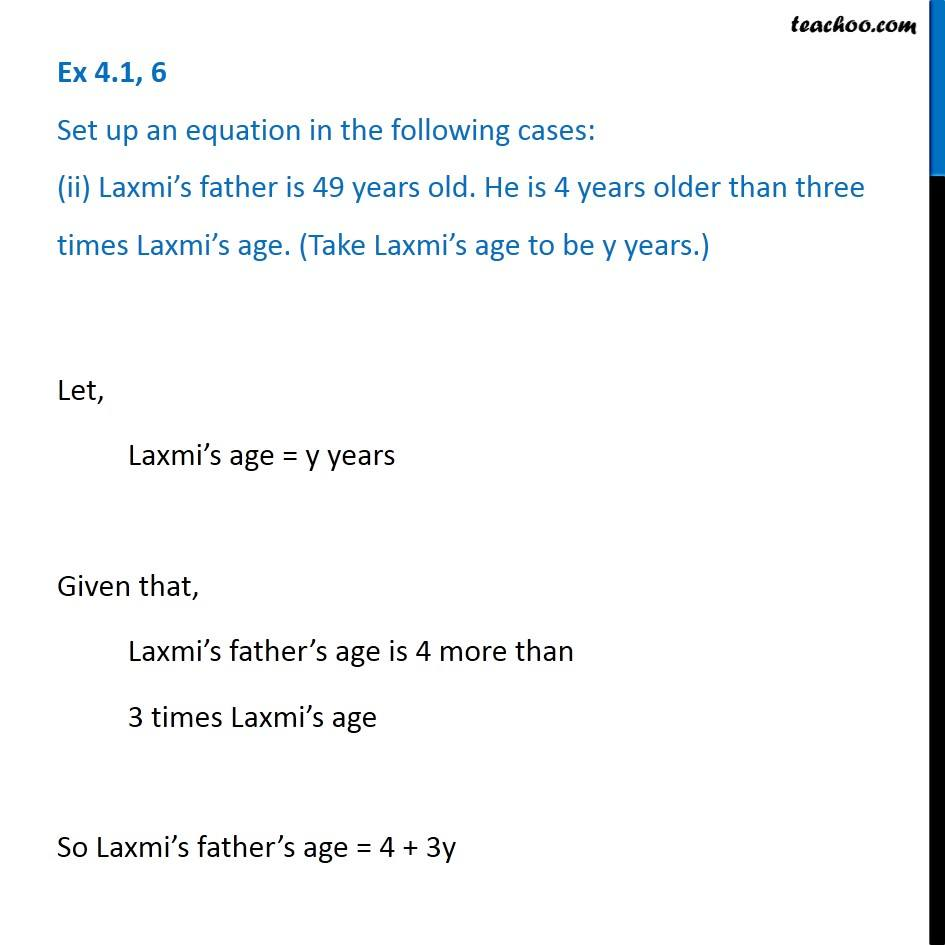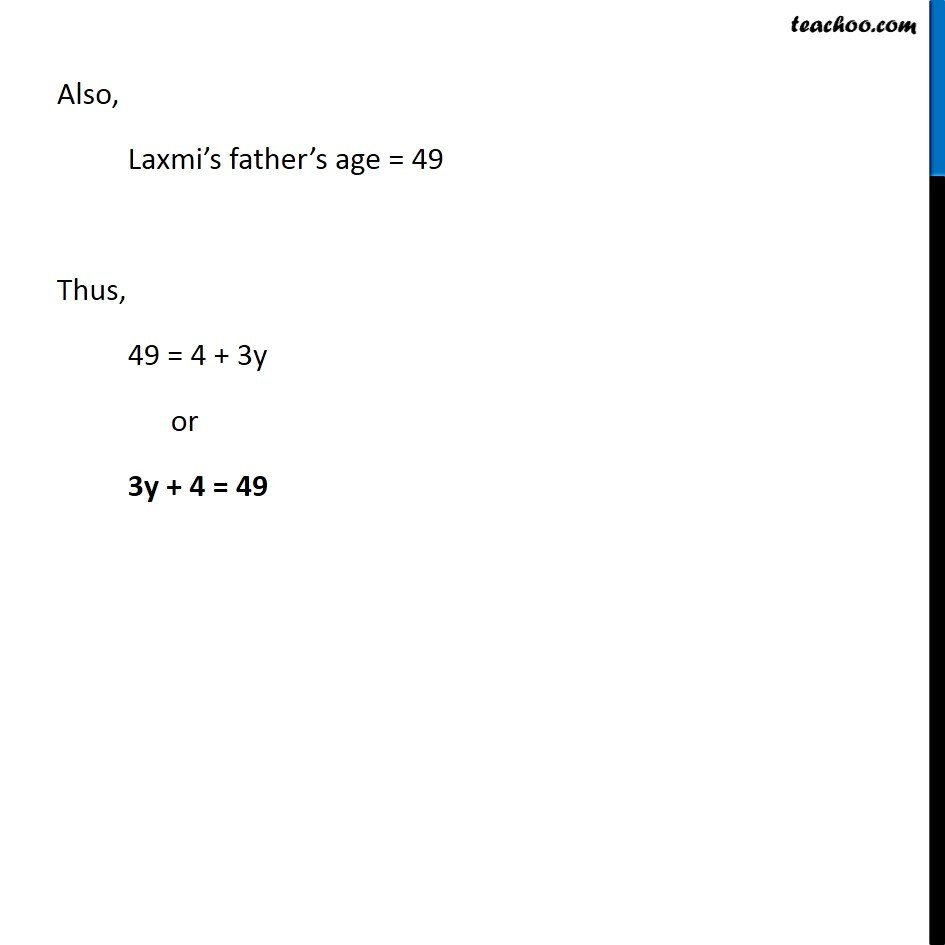Ex 4.1

Chapter 4 Class 7 Simple Equations
Serial order wiseIntroducing your new favourite teacher - Teachoo Black, at only ₹83 per month

### Transcript

Ex 4.1, 6 Set up an equation in the following cases: (ii) Laxmi s father is 49 years old. He is 4 years older than three times Laxmi s age. (Take Laxmi s age to be y years.)Let, Laxmi s age = y years Given that, Laxmi s father s age is 4 more than 3 times Laxmi s age So Laxmi s father s age = 4 + 3y Also, Laxmi s father s age = 49 Thus, 49 = 4 + 3y or 3y + 4 = 49